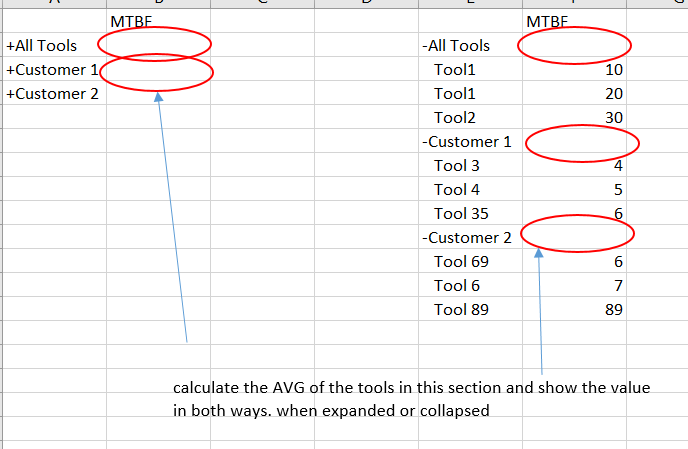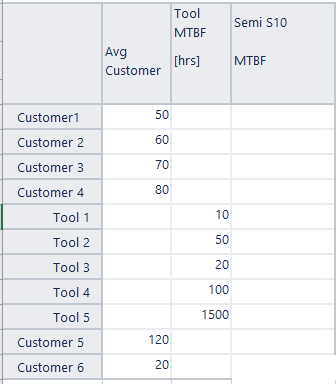# Calculate Average instead of Sum

Dear EazyBI Community,

i tried now for weeks to get this running but i am failing.

I have a Dimension called Tools and this includes for every tool i have one entry.
I calculate the MTBF for each tool based on tickets.
What i need is the average of the tools in collapsed and expanded view.Calculation of MTBF:
Case when [Tool].CurrentHierarchyMember.Name not MATCHES “TSMC MBMW Fleet”
THEN
CASE WHEN DateInPeriod(Now(), [Time].CurrentHierarchyMember)
THEN
IIf([Measures].[Incidents]>0,DateDiffDays([Time].[Year].CurrentDateMember.StartDate, DateWithoutTime(Now())) * 24 /
[Measures].[Incidents],DateDiffDays([Time].[Year].CurrentDateMember.StartDate, DateWithoutTime(Now())) * 24 / 1)

``````  ELSE DateDiffDays([Time].CurrentMember.StartDate, [Time].CurrentMember.NextStartDate) * 24 /
[Measures].[Incidents]
END
``````

END

Calculation of MTBF AVG
CASE WHEN [Tool].CurrentHierarchyMember.Name = “TSMC MBMW Fleet” THEN
Avg(
–set of issues with lead time in selected period
Filter(
Descendants([Tool].CurrentHierarchyMember, [Tool].[Tool]),
[Measures].[MTBF [hrs]]]> 0),
–for those issues calcaulte the average lead time
[Measures].[MTBF [hrs]]]
)
END

But i dont get any value here.
BR, Herbert

The interesting part is that with the pre defined “All Tools” Hirarchy it is working! Only for my defined calculated members in Tools it is not working.

Here the calculated member Tools for Customer 1:
Aggregate(
Filter(
[Tool].Members,
[Tool].CurrentMember.Name MATCHES ‘TC.*’
)
)

Hi @HeWe,

The key here is that you have the Aggregate(…) members in the Tools dimension. Instead of `Descendants()`, you would use the DescendantsSet():

``````Avg(
--set of issues with lead time in the selected period
Filter(
DescendantsSet([Tool].CurrentHierarchyMember, [Tool].[Tool]),
[Measures].[MTBF [hrs]]]> 0),
-- for those issues calcaulte the average lead time
[Measures].[MTBF [hrs]]]
)
``````

To check the set over which the calculation is performed, you can use the SetToStr() function:

``````SetToStr(Filter(
Descendants([Tool].CurrentHierarchyMember, [Tool].[Tool]),
[Measures].[MTBF [hrs]]]> 0)
)
``````

or

``````SetToStr(Filter(
DescendantsSet([Tool].CurrentHierarchyMember, [Tool].[Tool]),
[Measures].[MTBF [hrs]]]> 0)
)
``````

Additionally, I would like to point out that instead of aggregated members in the Tools dimension, you might check out building a custom hierarchy in the dimension.

Lauma / support@eazybi.com

Hi Lauma.cirule

thanks for your help. This solves my issue.
Correlating to this i need some small help.
When i calculate the MTBF it is designed to calculate the MTBF on Tool level.

As you can see in the MTBF i filter for Customer 1. How can i change this that for every Customer this is not calculate? Or shall i just add: Case when [Tool].CurrentHierarchyMember.Name not MATCHES „Customer 1 or Customer 2 or Customer 3. Or can i filter here for a hirarchy level?

Hi @HeWe,

Yes, you can get the level name and filter for that. E.g., that it is only calculated on level `Tool`

``````CASE WHEN [Tool].CurrentMember.Level.Name MATCHES "Tool"
...
``````

Lauma / support@eazybi.com

Dear Lauma,

thanks a lot.
That worked fine.
I only have now one last topic for this questions.
I am currently calculating the AVG for each Customer and this is working.
I need another calculation which removes the tool per customer with the maximum and minimum MTFB.
It worked before i used the DecendantsSet.
Here to code i have
ASE WHEN [Tool].CurrentHierarchyMember.Name MATCHES “TSMC MBMW Fleet|IMO MBMW Fleet|SK Hynix MBMW Fleet|SEC MBMW Fleet|DNP MBMW Fleet” THEN
Avg(
Filter(
DescendantsSet([Tool].CurrentHierarchyMember, [Tool].[Tool]),
[Measures].[Tool MTBF [hrs]]]<>
Max(DescendantsSet([Tool].CurrentHierarchyMember, [Tool].[Tool]),[Measures].[Tool MTBF [hrs]]])
AND
[Measures].[Tool MTBF [hrs]]]<>
Min(DescendantsSet([Tool].CurrentHierarchyMember, [Tool].[Tool]),[Measures].[Tool MTBF [hrs]]])
),
[Measures].[Tool MTBF [hrs]]]
)
But with Decendends Set it is not working.

The simple AVG is working:
Avg(
–set of issues with lead time in selected period
Filter(
DescendantsSet([Tool].CurrentHierarchyMember, [Tool].[Tool]),
[Measures].[Tool MTBF [hrs]]]> 0),
–for those issues calcaulte the average lead time
[Measures].[Tool MTBF [hrs]]]
)

Hi @HeWe,

Could you please add more details of what is not working? For example, is the formula giving an unexpected result or some error?
A screenshot or report definition would be helpful. You may send it over to support@eazybi.com.

Lauma / support@eazybi.com

Hi Lauma,

no error in the formular. The field is just empty.Currently i just like to remove the lowest number from the average:
Avg(
Filter(
DescendantsSet([Tool].CurrentHierarchyMember, [Tool].[Tool]),
[Measures].[Tool MTBF [hrs]]]<>
Min(DescendantsSet([Tool].CurrentHierarchyMember, [Tool].[Tool]),[Measures].[Tool MTBF [hrs]]]))
,[Measures].[Tool MTBF [hrs]]]
)

Hi @HeWe,

I see. And the problem is that the formula is written so that it does not take the min and max value from “neighbours”, but from the tool itself filtering themselves out.

Please try the following formula - it would show the value on the Customer level, taking out the min and max tool from the avg:

``````Avg(
BottomCount(
TopCount(
Filter(
DescendantsSet([Tool].CurrentHierarchyMember, [Tool].[Tool]),
[Measures].[Tool MTBF [hrs]]] > 0),
Count(DescendantsSet([Tool].CurrentHierarchyMember, [Tool].[Tool]))-1,
[Measures].[Tool MTBF [hrs]]]
), Count(DescendantsSet([Tool].CurrentHierarchyMember, [Tool].[Tool]))-2,
[Measures].[Tool MTBF [hrs]]]
), [Measures].[Tool MTBF [hrs]]]
)
``````

Lauma / support@eazybi.com

You are just awsome - thank you so mucht!!!

1 Like